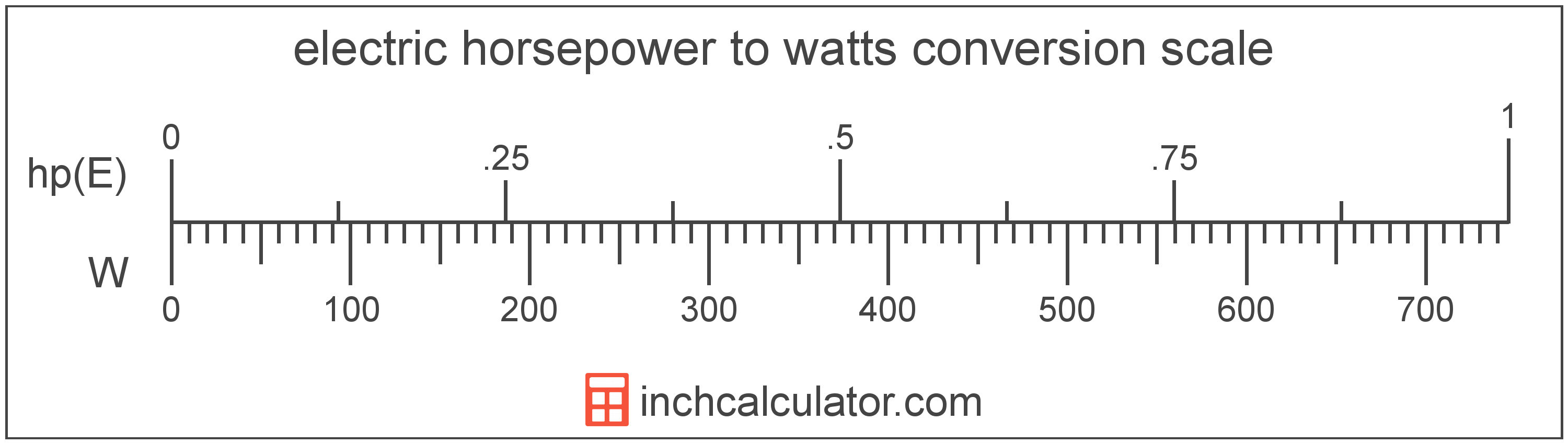# Convert Electric Horsepower to Watts

Enter the power in electric horsepower below to get the value converted to watts.

Results in Watts:1 hp(E) = 746 W

## How to Convert Electric Horsepower to WattsTo convert a electric horsepower measurement to a watt measurement, multiply the power by the conversion ratio. One electric horsepower is equal to 746 watts, so use this simple formula to convert:

watts = electric horsepower × 746

The power in watts is equal to the electric horsepower multiplied by 746.

For example, here's how to convert 5 electric horsepower to watts using the formula above.
5 hp(E) = (5 × 746) = 3,730 W

Electric horsepower and watts are both units used to measure power. Keep reading to learn more about each unit of measure.

## Electric Horsepower

Electric horsepower is used to measure the output of electric motors and other electrical machines in the US. One electric horsepower is equal to exactly 746 watts.

The electric horsepower is a US customary and imperial unit of power. Electric horsepower can be abbreviated as hp(E), for example 1 electric horsepower can be written as 1 hp(E).

## Watts

One watt is the power equal to the rate of energy of one joule per second in an electrical circuit. One watt is also equal to the power produced in a circuit with a voltage potential of one volt with a current of one amp.

The watt is the SI derived unit for power in the metric system. Watts can be abbreviated as W, for example 1 watt can be written as 1 W.

Watt's Law states the relationship between power, current, and voltage. Using Watt's Law, it's possible to express the power in watts as an expression using current and voltage.

PW = IA × VV

The power in watts is equal to the current in amperes times the potential difference in volts.

Combining this with Ohm's Law, it's also possible to express the power in watts using resistance in addition to volts and amps.

## Electric Horsepower Measurements and Equivalent Watt Conversions

Common electric horsepower values converted to the equivalent watt value
Electric Horsepower Watts
1 hp(E) 746 W
2 hp(E) 1,492 W
3 hp(E) 2,238 W
4 hp(E) 2,984 W
5 hp(E) 3,730 W
6 hp(E) 4,476 W
7 hp(E) 5,222 W
8 hp(E) 5,968 W
9 hp(E) 6,714 W
10 hp(E) 7,460 W
11 hp(E) 8,206 W
12 hp(E) 8,952 W
13 hp(E) 9,698 W
14 hp(E) 10,444 W
15 hp(E) 11,190 W
16 hp(E) 11,936 W
17 hp(E) 12,682 W
18 hp(E) 13,428 W
19 hp(E) 14,174 W
20 hp(E) 14,920 W
21 hp(E) 15,666 W
22 hp(E) 16,412 W
23 hp(E) 17,158 W
24 hp(E) 17,904 W
25 hp(E) 18,650 W
26 hp(E) 19,396 W
27 hp(E) 20,142 W
28 hp(E) 20,888 W
29 hp(E) 21,634 W
30 hp(E) 22,380 W
31 hp(E) 23,126 W
32 hp(E) 23,872 W
33 hp(E) 24,618 W
34 hp(E) 25,364 W
35 hp(E) 26,110 W
36 hp(E) 26,856 W
37 hp(E) 27,602 W
38 hp(E) 28,348 W
39 hp(E) 29,094 W
40 hp(E) 29,840 W

## More Electric Horsepower Power Conversions

SI Units
Convert to Microwatts
1 hp(E) is equal to 746,000,000 microwatts
Convert to Milliwatts
1 hp(E) is equal to 746,000 milliwatts
Convert to Kilowatts
1 hp(E) is equal to 0.746 kilowatts
Convert to Megawatts
1 hp(E) is equal to 0.000746 megawatts
US Customary & Imperial Units
Convert to Mechanical Horsepower
1 hp(E) is equal to 1.000402 mechanical horsepower
Convert to Btu Per Hour
1 hp(E) is equal to 2,545.46 btu per hour
Other Units
Convert to Kilocalories Per Hour
1 hp(E) is equal to 641.873928 kilocalories per hour